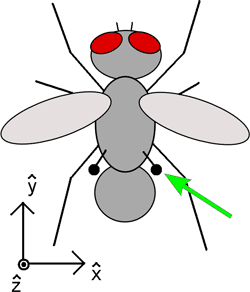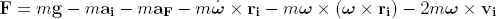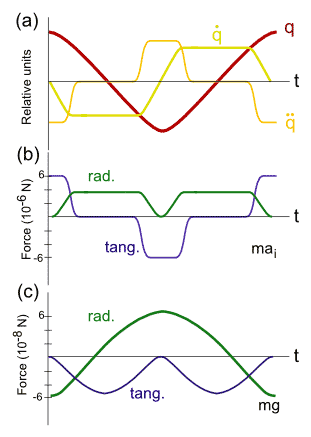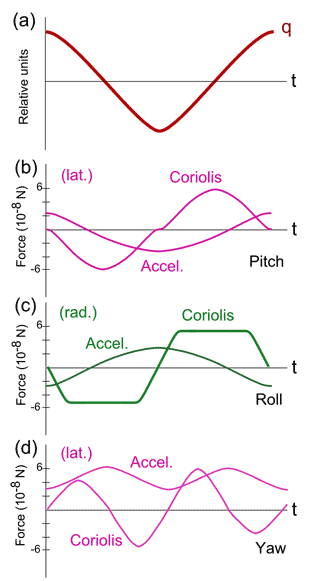Halteres: Mechanisms for Stable Insect Flight

Jonathan A. Sobota December 10, 2007

(Submitted as coursework for Physics 210, Stanford University, Fall 2007)Fig. 1: Schematic illustration of a fly, with the position of the right haltere indicated with a green arrow. A coordinate system is defined for the following discussion.

Introduction

Even a casual observation of a fly's flight will reveal that is a remarkably maneuverable animal. Indeed, studies have shown that a male housefly can initiate a corrective tracking maneuver within 30 ms of a change in the trajectory of the female that it is pursuing. Therefore, it is not surprising that a great deal of research concentrates on the question of how flies achieve such agile flight. Clearly, the fly's wings are essential for controlling its flight pattern, and its eyes provide visual feedback. However, a third, less obvious, but equally important, mechanism is provided by a pair of small, club-like organs known as halteres.

The approximate size, shape, and location of the halteres are indicated in Fig. 1. Each haltere consists of a massive tip, known as the knob, connected to the body by a thin rod, known as the stalk. It is believed that the halteres evolved from a pair of hind wings. They beat in antiphase to the wings, but due to their small dimensions, it is clear that they serve no aerodynamical purpose. The base of the stalk is rich with sensory cells, suggesting that the halteres serve as sensory organs. Indeed, it has been found that the primary function of halteres is to provide the fly with information regarding its angular velocity and angular acceleration through space . When the halteres are cut off or immobilized, the fly spins around and falls to the ground, which serves as evidence that this sensory feedback is essential for maintaining stable flight . This article will review the dynamics of halteres. This will show, at least in principle, that the halteres are capable of providing valuable feedback to the fly.

Haltere Dynamics

We now analyze the forces acting on the halteres, following the approach of . We neglect the stalk in this discussion, and assume that all of the relevant physics can be understood from the forces on the knob. This assumption is justified by the fact that most of the haltere's mass is concentrated in the knob, and that the knob moves at higher relative velocities as compared to the stalk. We write the effective force on the knob F as:Fig. 2: (a) The angular displacement q of the haltere over one period of oscillation. The dots represent derivatives with respect to time. (b) The radial and tangential components of the primary force. No lateral components are present. (c) The radial and tangential components of the gravitational force. No lateral components are present for a horizontal fly. Note that these graphs are meant to depict the important qualitative features, but are not necessarily quantitatively accurate.

where m is the mass of the knob, g is the gravitational acceleration, ri, vi, and ai are the position, velocity, and acceleration of the knob relative to the fly, aF is the translational acceleration of the fly relative to an inertial reference frame, and &omega is the angular velocity of the fly. The first term is simply the gravitational force on the knob. The second term, which we call the primary force, is due to the acceleration of the knob relative to the fly. The third and fourth terms are due to the translational acceleration and angular acceleration of the fly, respectively. The fifth and sixth terms represent the centrifugal force and the Coriolis force, respectively.

To discuss these forces in more detail, we establish the x, y, and z directions as illustrated in Fig. 1, with g directed along the -z direction. It has been observed that each haltere oscillates in a vertical plane tilted 30 degrees towards the back of the insect. They oscillate with a frequency of 110 to 180 Hz in antiphase to the oscillation of the wings. Over one oscillation period, the halteres sweep out an angular range of ~170 degrees. It has further been observed that the angular velocity is nearly constant in magnitude. This behavior is sketched schematically in Fig. 2a, where we define q as the angular displacement of the haltere. We note that the mass of the knob is known to be approximately m = 4.75 μg, and the length of the stalk is approximately 1.07 mm. Using this data, we can begin to quantitatively evaluate the contributions of each of the forces described above.

We consider the forces in three orthogonal components: the radial and tangential components are defined as usual, with the radial component directed along the stalk, and the tangential component directed perpendicular to the radial component within the plane of oscillation. The third component, directed perpendicular to the plane of oscillation, we define as the lateral component.

The primary and gravitational forces are sketched schematically in Fig. 2b and c for an insect oriented horizontally. We note that these forces have no lateral components. We see that the gravitational force is smaller than the primary force by two orders of magnitude, which makes it unlikely that the insect would be able to detect the gravitational force. Therefore, we conclude that the insect gets no information about the direction of g from its halteres.Fig. 3: Coriolis and angular acceleration forces for &omega = 320 deg/s and dω/dt = .26 deg/(ms)2 for rotations/accelerations about each axis. (a) The angular displacement q of the haltere over one period of oscillation (identical to that depicted in Fig. 2a). (b) For rotations and accelerations about the x-axis (pitch), the forces act in the lateral direction. (c) For rotations and accelerations about the y-axis (roll), the forces act in the radial direction. (d) For rotations and accelerations about the z-axis (yaw), the forces act in the lateral direction.

Next we consider the forces dependent on angular velocity and angular acceleration. We define pitch, roll, and yaw as rotations about the x, y, and z axes, respectively. To evalauate these forces, we choose fixed magnitudes of &omega and dω/dt and then plot the resulting forces for rotations and accelerations about all three axes. The resulting Coriolis and angular acceleration forces for &omega = 320 deg/s and dω/dt = .26 deg/(ms)2 are sketched in Fig. 3b, c, and d for pitch, roll, and yaw, respectively. For reference, the haltere angular displacement q is again plotted in Fig. 3a. Several observations can be made from these plots. First, rotations and accelerations about the y-axis (roll) produce radial forces of order 6x10-2 μN. From Fig. 2b, we see that this is a factor of 100 smaller than the radial component of the primary force. Therefore, it is unlikely that the insect is capable of roll detection through its halteres. However, we observe that the pitch and yaw responses are entirely in the lateral direction, making pitch and yaw detection possible. We note that the pitch response has the same frequency as the haltere's beating frequency, whereas the yaw response has double the frequency. Furthermore, we observe that the Coriolis and angular acceleration forces are out of phase by 90 degrees, making it possible to differentiate between angular velocities and accelerations.

In the above discussion of angular forces, we neglected to include the centrifugal force. This is because the centrifugal force is smaller than the Coriolis force by a factor of (dq/dt)/ω, which is usually on the order of 30 to 3000, therefore making detection unlikely. Furthermore, the centrifugal force depends on the square of ω, so even if this force was detectable, it would not contribute to a directional response.

Conclusion

This article has illustrated the physical principles by which halteres can detect angular velocities and angular accelerations of the fly. However, this is far from an exhaustive study of haltere function. A vast amount of research utilizing innovative experimental techniques has been done, shedding light on how flies collect, process, and respond to the feedback provided by the halteres . This is beyond the scope of this article, and the interested reader is advised to consult the references for a detailed study of this fascinating topic.

References

 W. P. Chan, F. Prete and M. H. Dickinson, Science 280, 289 (1998).

 G. Nalbach, J. Comp. Physiol. A 173, 293 (1993).

 G. Nalbach, Neuroscience 61, 149 (1994).